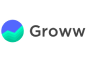New update is available. Click here to update.
Topics

# Ways To Make Coin Change

Moderate0/80
Average time to solve is 20m+65 more companies

## Problem statement

You are given an infinite supply of coins of each of denominations D = {D0, D1, D2, D3, ...... Dn-1}. You need to figure out the total number of ways W, in which you can make a change for value V using coins of denominations from D. Print 0, if a change isn't possible.

Detailed explanation ( Input/output format, Notes, Images )
Sample Input 1 :
``````3
1 2 3
4
``````
Sample Output 1:
``````4
``````
Explanation for Sample Output 1:
``````We can make a change for the value V = 4 in four ways.
1. (1,1,1,1),
2. (1,1, 2), [One thing to note here is, (1, 1, 2) is same as that of (2, 1, 1) and (1, 2, 1)]
3. (1, 3), and
4. (2, 2)
``````
Sample Input 2 :
``````3
5 3 2
1
``````
Sample Output 2:
``````0
``````Console